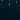# Higher order functions

I recently started learning JavaScript and I tumbled upon a concept called Higher Order Functions (HOF). There are quite a lot of documents in the internet that explains what an HOF is, and here is my thought.

Before explaining what an HOF is, let’s see how a function looks like in JavaScript. Functions in JavaScript is not different from functions in any other programming languages, which means, I assume that you kinda know how a function generally looks like. Below is a function snippet in JavaScript.

function myTestFunction() {
}

Now, that we know how a function looks like in JavaScript, let’s continue on knowing what HOF is and how is it different from a normal function.

HOF is a concept in JavaScript where a function (say x) is passed as an argument to another function (say y) OR the calling function (say z) returns a function (say x) after execution

Let’s take the above function for example (#1), and pass it into another function

document.addEventListener("click", myTestFunction)
function myTestFunction() {
}

Now, document.addEventListener is called whenever an event in the first parameter is triggered in the document, and the second parameter is a function that gets executed whenever the event is triggered

In other words, the above function can also be written as

document.addEventListener("click", function myTestFunction() {
})

And this works perfectly fine. So, if this works perfectly fine, why would I need to write another function and call it within the addEventListener instead of writing directly within the listener. The answer is, the function can be reused now, if it’s outside the listener. Kinda makes sense?

Let’s take another step and look at another example (#2) which is a little more complex.

function createMultiplier(multiplier) {
return function(x) {
return x * multiplier
}
}

let doubleMe = createMultiplier(2)
let tripleMe = createMultiplier(3)

document.write(doubleMe(45) + "<br>")
document.write(tripleMe(45) + "<br>")
document.write(quadrupleMe(45) + "<br>")

As you can see, the createMultiplier function returns a function here which can be used in different places. Let’s take a look at how the code looks without HOF

function multiply(x, multiplier) {
return x * multiplier
}

let doubleMe = multiply(45, 2)
let tripleMe = multiply(45, 3)

document.write(doubleMe + "<br>")
document.write(tripleMe + "<br>")
document.write(quadrupleMe + "<br>")

Looks like, the code without HOF is still less number of lines and effective too! Until now, even I was not sure about the use of HOF. But let’s take 1 more step from here.

Let’s parameterize the multiplier (#3)

### With HOF

function createMultiplier(multiplier) {
return function(x) {
return x * multiplier
}
}

let nums = [41, 42, 43, 44, 45, 46]

let doubleMe = createMultiplier(2)
let tripleMe = createMultiplier(3)

for (i = 0; i < nums.length; i++) {
document.write(doubleMe(nums[i]) + "<br>")
document.write(tripleMe(nums[i]) + "<br>")
}

### Without HOF

function multiply(x, multiplier) {
return x * multiplier
}

let nums = [41, 42, 43, 44, 45, 46]

for (i = 0; i < nums.length; i++) {
let doubleMe = multiply(nums[i], 2)
let tripleMe = multiply(nums[i], 3)

document.write(doubleMe + "<br>")
document.write(tripleMe + "<br>")
}

As you can see, the numbers 2, 3 and 4 are constants and we are not making any changes to those parameters within the loop. However, we cannot move them outside of the for loop since the resultant value is dependent on this constant.

Whereas, in the code with HOF, we have moved the constants successfully outside of the loop. Now, why do we do that? Does it impact the performance?

The answer in this case is, the code looks a little bit neat and NO it does not affect the performance.

Here’s another example of HOF in JavaScript

let myColors = ["red", "orange", "yellow", "green"]
myColors.forEach(saySomethingNice)
function saySomethingNice(color) {
document.write("The color " + color + " is a great color. <br>")
}

The forEach method in the array object automatically loops thro’ every element in the array without using a loop, which means less code to do the same work.

The code in example #3 looks like this now!

function createMultiplier(multiplier) {
return function(x) {
return x * multiplier
}
}

let nums = [41, 42, 43, 44, 45, 46]

let doubleMe = createMultiplier(2)
let tripleMe = createMultiplier(3)

nums.forEach(num => {
document.write(doubleMe(num) + "<br>")
document.write(tripleMe(num) + "<br>")
})